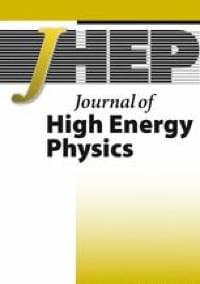We discuss the possibility to predict the QCD axion mass in the context of grand unified theories. We investigate the implementation of the DFSZ mechanism in the context of renormalizable SU theories. In the simplest theory, the axion mass can be predicted with good precision in the range ma = (2–16) neV, and there is a strong correlation between the predictions for the axion mass and proton decay rates. In this context, we predict an upper bound for the proton decay channels with antineutrinos, τ p → K + ν ¯ ≲ 4 × 10 37 $$\tau \left(p\to {K}^{+}\overline{ u}\right)\lesssim 4\times {10}^{37}$$ yr and τ p → π + ν ¯ ≲ 2 × 10 36 $$\tau \left(p\to {\pi}^{+}\overline{ u}\right)\lesssim 2\times {10}^{36}$$ yr. This theory can be considered as the minimal realistic grand unified theory with the DFSZ mechanism and it can be fully tested by proton decay and axion experiments.